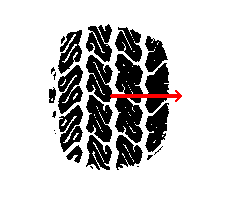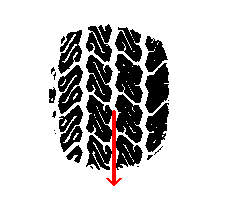# Numb3rs 403: Velocity

In this episode, street racers crash into a local coffee shop, killing one person and injuring several others. As Don and the gang investigate, Charlie develops a mathematical model of the accident on the computer to recreate the path that the car took just before the accident. One important component of that model is the friction circle.

## Mathematical Models

Contrary to its name, model theory, is not the study of mathematical models. Rather, it is a branch of logic that is similar in nature to abstract algebra.

Mathematical models are important tools in physics, engineering, biology, economics, sociology, and many other disciplines. The goal of a model is to use math to explain the behavior of an observed phenomenon such that the model behavior coincides with the observed behavior. We can also use these models in a predictive fashion and form hypotheses about future behavior of the phenomenon. The better our model is, the more accurate our hypotheses will be.

You may already be familiar with quite a few mathematical models. For instance, the equations one derives in mechanics are models for Newton's laws of motion. Graphs of supply and demand curves in economics are models of the economy. These models rely mostly on Calculus. There are, however, many other models out there that use very different kinds of math to calculus. For example, many social scientists use game theory to build models because it lends itself to well to decision based phenomenon. In fact, in the show, Charlie is writing a book which uses game theory to describe friendship and personal relationships. Other areas of modeling including using group theory in chemistry and algebraic geometry in biology.

## Friction Circle

Part of the model that Charlie builds to reconstruct the accident involves the concept of the friction circle. This idea has to do specifically with how cars maintain traction with the road while driving, that is, the force of friction between the road and the tires.

We begin by recalling that the force of friction, F, acting on an object has magnitude F = &mu*|N| where N is the normal force (or weight) of the object. The direction of the frictional force depends on the direction of motion. For example, the image on the left shows the frictional force if the car moving laterally to the left and the picture on the right shows the frictional force if the car was heading straight forward:Since a car can drive in any direction, we can have a frictional force pointing in any direction. So long as the other forces acting on the car don't exceed the force of friction, we'll maintain traction. Thus, we have a friction circle of radius &mu*|N|, where our car will maintain traction with the road, regardless of the direction of motion.

This becomes particularly important if we are racing and want to be able to accelerate or decelerate through a turn. For instance, the diagram below shows the forces if we try to break while heading in to a left hand turn.As we can see, the resulting frictional force is the sum of the breaking and turning frictional forces. This resulting frictional force is the one that must stay inside the friction circle in order for us to keep from sliding.

Much of what has been said here has come from this website, which is far more in depth and a great resource for those wanting to read more. Specifically, we extend our thanks for the friction circle images included here.